Home > PC > Chapter 11 > Lesson 11.1.2 > Problem11-23

11-23.
1.In the diagram at right, the point P has Cartesian coordinates (x, y) and polar coordinates (r, θ). Homework Help ✎

1. Explain why x = r cosθ.

2. Find y in terms of r and θ.

3. Find r in terms of x and y.

4. Find θ in terms of x and y.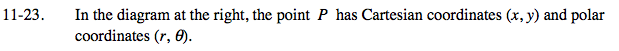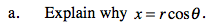Using the diagram, cos(θ) = x/r.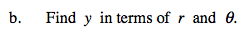Using the diagram, sin(θ) = ?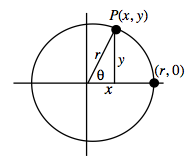What theorem can be used to relate the three sides of a right triangle?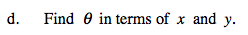Use tangent.